# Fast.ai v3 2019 part 1 lesson 1 dictation

One of Jeremy’s advices EN 中文 is to write all the notebooks from scratch. Maybe dictation as the following is one small step towards putting this advice into practice.

# Lesson 1 - What’s your pet

the purpose of this Nb

#### the purpose of this Nb

In this lesson we will build our first image classifier from scratch, and see if we can achieve world-class results. Let’s dive in!

three lines of magics

#### three lines of magics

Every notebook starts with the following three lines; they ensure that any edits to libraries you make are reloaded here automatically, and also that any charts or images displayed are shown in this notebook.

``````%reload_ext autoreload
%matplotlib inline
``````
how fastai designs import

#### how fastai designs import

We import all the necessary packages. We are going to work with the fastai V1 library which sits on top of Pytorch 1.0. The fastai library provides many useful functions that enable us to quickly and easily build neural networks and train our models.

import everything we need

#### import everything we need

``````from fastai.vision import *
from fastai.metrics import error_rate
``````
how to handle out of memory problem?

#### how to handle out of memory problem?

If you’re using a computer with an unusually small GPU, you may get an out of memory error when running this notebook. If this happens, click Kernel->Restart, uncomment the 2nd line below to use a smaller batch size (you’ll learn all about what this means during the course), and try again.

set batch_size

#### set batch_size

``````bs = 64
# bs = 16   # uncomment this line if you run out of memory even after clicking Kernel->Restart
``````

## Looking at the data

#### What Pets dataset is about?

We are going to use the Oxford-IIIT Pet Dataset by O. M. Parkhi et al., 2012 which features 12 cat breeds and 25 dogs breeds. Our model will need to learn to differentiate between these 37 distinct categories. According to their paper, the best accuracy they could get in 2012 was 59.21%, using a complex model that was specific to pet detection, with separate “Image”, “Head”, and “Body” models for the pet photos. Let’s see how accurate we can be using deep learning!

We are going to use the `untar_data` function to which we must pass a URL as an argument and which will download and extract the data.

How to get docs

#### How to get docs

``````help(untar_data)
``````
``````Help on function untar_data in module fastai.datasets:

untar_data(url: str, fname: Union[pathlib.Path, str] = None, dest: Union[pathlib.Path, str] = None, data=True, force_download=False) -> pathlib.Path
Download `url` to `fname` if it doesn't exist, and un-tgz to folder `dest`.
``````
how fastai get dataset

#### how fastai get dataset

``````path = untar_data(URLs.PETS); path
``````
``````PosixPath('/home/ubuntu/.fastai/data/oxford-iiit-pet')
``````
how to see inside a folder

#### how to see inside a folder

``````path.ls()
``````
``````[PosixPath('/home/ubuntu/.fastai/data/oxford-iiit-pet/images'),
PosixPath('/home/ubuntu/.fastai/data/oxford-iiit-pet/annotations')]
``````
how to build path to sub-folders

#### how to build path to sub-folders

``````path_anno = path/'annotations'
path_img = path/'images'
``````
what does it mean to look at the data

#### what does it mean to look at the data

The first thing we do when we approach a problem is to take a look at the data. We always need to understand very well what the problem is and what the data looks like before we can figure out how to solve it. Taking a look at the data means understanding how the data directories are structured, what the labels are and what some sample images look like.

getting labels is the key of handling dataset

#### getting labels is the key of handling dataset

The main difference between the handling of image classification datasets is the way labels are stored. In this particular dataset, labels are stored in the filenames themselves. We will need to extract them to be able to classify the images into the correct categories. Fortunately, the fastai library has a handy function made exactly for this, `ImageDataBunch.from_name_re` gets the labels from the filenames using a regular expression.

turn files inside a folder into a list of path objects

#### turn files inside a folder into a list of path objects

``````fnames = get_image_files(path_img)
fnames[:5]
``````
``````[PosixPath('/home/ubuntu/.fastai/data/oxford-iiit-pet/images/saint_bernard_188.jpg'),
PosixPath('/home/ubuntu/.fastai/data/oxford-iiit-pet/images/staffordshire_bull_terrier_114.jpg'),
PosixPath('/home/ubuntu/.fastai/data/oxford-iiit-pet/images/Persian_144.jpg'),
PosixPath('/home/ubuntu/.fastai/data/oxford-iiit-pet/images/Maine_Coon_268.jpg'),
PosixPath('/home/ubuntu/.fastai/data/oxford-iiit-pet/images/newfoundland_95.jpg')]
``````
how to make sure the same validation set?

#### how to make sure the same validation set?

``````np.random.seed(2)
pat = r'/([^/]+)_\d+.jpg\$'
``````
how to create an ImageDataBunch from re

#### how to create an ImageDataBunch from re

``````data = ImageDataBunch.from_name_re(path_img,
fnames,
pat,
ds_tfms=get_transforms(),
size=224,
bs=bs
).normalize(imagenet_stats)
``````
print out images with labels

#### print out images with labels

``````data.show_batch(rows=3, figsize=(7,6))
``````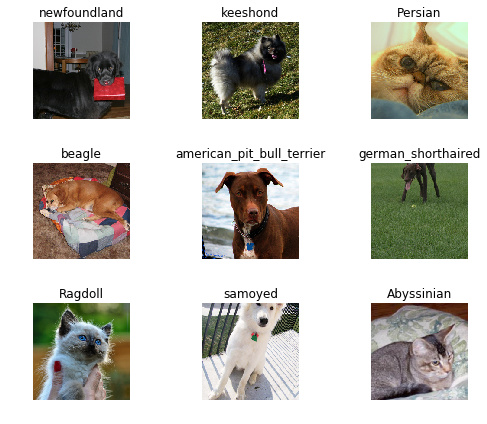print out all classes and c

#### print out all classes and c

``````print(data.classes)
len(data.classes),data.c
``````
``````['Abyssinian', 'Bengal', 'Birman', 'Bombay', 'British_Shorthair', 'Egyptian_Mau', 'Maine_Coon', 'Persian', 'Ragdoll', 'Russian_Blue', 'Siamese', 'Sphynx', 'american_bulldog', 'american_pit_bull_terrier', 'basset_hound', 'beagle', 'boxer', 'chihuahua', 'english_cocker_spaniel', 'english_setter', 'german_shorthaired', 'great_pyrenees', 'havanese', 'japanese_chin', 'keeshond', 'leonberger', 'miniature_pinscher', 'newfoundland', 'pomeranian', 'pug', 'saint_bernard', 'samoyed', 'scottish_terrier', 'shiba_inu', 'staffordshire_bull_terrier', 'wheaten_terrier', 'yorkshire_terrier']
``````
``````(37, 37)
``````

## Training: resnet34

what is transfer learning like?

#### what is transfer learning like?

Now we will start training our model. We will use a convolutional neural network backbone and a fully connected head with a single hidden layer as a classifier. Don’t know what these things mean? Not to worry, we will dive deeper in the coming lessons. For the moment you need to know that we are building a model which will take images as input and will output the predicted probability for each of the categories (in this case, it will have 37 outputs).

We will train for 4 epochs (4 cycles through all our data).

how to create a CNN model as transfer learning

#### how to create a CNN model as transfer learning

``````learn = create_cnn(data, models.resnet34, metrics=error_rate)
``````
how to see the structure of model

#### how to see the structure of model

``````learn.model
``````
``````Sequential(
(0): Sequential(
(0): Conv2d(3, 64, kernel_size=(7, 7), stride=(2, 2), padding=(3, 3), bias=False)
(1): BatchNorm2d(64, eps=1e-05, momentum=0.1, affine=True, track_running_stats=True)
(2): ReLU(inplace)
(3): MaxPool2d(kernel_size=3, stride=2, padding=1, dilation=1, ceil_mode=False)
(4): Sequential(
(0): BasicBlock(
(conv1): Conv2d(64, 64, kernel_size=(3, 3), stride=(1, 1), padding=(1, 1), bias=False)
(bn1): BatchNorm2d(64, eps=1e-05, momentum=0.1, affine=True, track_running_stats=True)
(relu): ReLU(inplace)
(conv2): Conv2d(64, 64, kernel_size=(3, 3), stride=(1, 1), padding=(1, 1), bias=False)
(bn2): BatchNorm2d(64, eps=1e-05, momentum=0.1, affine=True, track_running_stats=True)
)
(1): BasicBlock(
(conv1): Conv2d(64, 64, kernel_size=(3, 3), stride=(1, 1), padding=(1, 1), bias=False)
(bn1): BatchNorm2d(64, eps=1e-05, momentum=0.1, affine=True, track_running_stats=True)
(relu): ReLU(inplace)
(conv2): Conv2d(64, 64, kernel_size=(3, 3), stride=(1, 1), padding=(1, 1), bias=False)
(bn2): BatchNorm2d(64, eps=1e-05, momentum=0.1, affine=True, track_running_stats=True)
)
(2): BasicBlock(
(conv1): Conv2d(64, 64, kernel_size=(3, 3), stride=(1, 1), padding=(1, 1), bias=False)
(bn1): BatchNorm2d(64, eps=1e-05, momentum=0.1, affine=True, track_running_stats=True)
(relu): ReLU(inplace)
(conv2): Conv2d(64, 64, kernel_size=(3, 3), stride=(1, 1), padding=(1, 1), bias=False)
(bn2): BatchNorm2d(64, eps=1e-05, momentum=0.1, affine=True, track_running_stats=True)
)
)
(5): Sequential(
(0): BasicBlock(
(conv1): Conv2d(64, 128, kernel_size=(3, 3), stride=(2, 2), padding=(1, 1), bias=False)
(bn1): BatchNorm2d(128, eps=1e-05, momentum=0.1, affine=True, track_running_stats=True)
(relu): ReLU(inplace)
(conv2): Conv2d(128, 128, kernel_size=(3, 3), stride=(1, 1), padding=(1, 1), bias=False)
(bn2): BatchNorm2d(128, eps=1e-05, momentum=0.1, affine=True, track_running_stats=True)
(downsample): Sequential(
(0): Conv2d(64, 128, kernel_size=(1, 1), stride=(2, 2), bias=False)
(1): BatchNorm2d(128, eps=1e-05, momentum=0.1, affine=True, track_running_stats=True)
)
)
(1): BasicBlock(
(conv1): Conv2d(128, 128, kernel_size=(3, 3), stride=(1, 1), padding=(1, 1), bias=False)
(bn1): BatchNorm2d(128, eps=1e-05, momentum=0.1, affine=True, track_running_stats=True)
(relu): ReLU(inplace)
(conv2): Conv2d(128, 128, kernel_size=(3, 3), stride=(1, 1), padding=(1, 1), bias=False)
(bn2): BatchNorm2d(128, eps=1e-05, momentum=0.1, affine=True, track_running_stats=True)
)
(2): BasicBlock(
(conv1): Conv2d(128, 128, kernel_size=(3, 3), stride=(1, 1), padding=(1, 1), bias=False)
(bn1): BatchNorm2d(128, eps=1e-05, momentum=0.1, affine=True, track_running_stats=True)
(relu): ReLU(inplace)
(conv2): Conv2d(128, 128, kernel_size=(3, 3), stride=(1, 1), padding=(1, 1), bias=False)
(bn2): BatchNorm2d(128, eps=1e-05, momentum=0.1, affine=True, track_running_stats=True)
)
(3): BasicBlock(
(conv1): Conv2d(128, 128, kernel_size=(3, 3), stride=(1, 1), padding=(1, 1), bias=False)
(bn1): BatchNorm2d(128, eps=1e-05, momentum=0.1, affine=True, track_running_stats=True)
(relu): ReLU(inplace)
(conv2): Conv2d(128, 128, kernel_size=(3, 3), stride=(1, 1), padding=(1, 1), bias=False)
(bn2): BatchNorm2d(128, eps=1e-05, momentum=0.1, affine=True, track_running_stats=True)
)
)
(6): Sequential(
(0): BasicBlock(
(conv1): Conv2d(128, 256, kernel_size=(3, 3), stride=(2, 2), padding=(1, 1), bias=False)
(bn1): BatchNorm2d(256, eps=1e-05, momentum=0.1, affine=True, track_running_stats=True)
(relu): ReLU(inplace)
(conv2): Conv2d(256, 256, kernel_size=(3, 3), stride=(1, 1), padding=(1, 1), bias=False)
(bn2): BatchNorm2d(256, eps=1e-05, momentum=0.1, affine=True, track_running_stats=True)
(downsample): Sequential(
(0): Conv2d(128, 256, kernel_size=(1, 1), stride=(2, 2), bias=False)
(1): BatchNorm2d(256, eps=1e-05, momentum=0.1, affine=True, track_running_stats=True)
)
)
(1): BasicBlock(
(conv1): Conv2d(256, 256, kernel_size=(3, 3), stride=(1, 1), padding=(1, 1), bias=False)
(bn1): BatchNorm2d(256, eps=1e-05, momentum=0.1, affine=True, track_running_stats=True)
(relu): ReLU(inplace)
(conv2): Conv2d(256, 256, kernel_size=(3, 3), stride=(1, 1), padding=(1, 1), bias=False)
(bn2): BatchNorm2d(256, eps=1e-05, momentum=0.1, affine=True, track_running_stats=True)
)
(2): BasicBlock(
(conv1): Conv2d(256, 256, kernel_size=(3, 3), stride=(1, 1), padding=(1, 1), bias=False)
(bn1): BatchNorm2d(256, eps=1e-05, momentum=0.1, affine=True, track_running_stats=True)
(relu): ReLU(inplace)
(conv2): Conv2d(256, 256, kernel_size=(3, 3), stride=(1, 1), padding=(1, 1), bias=False)
(bn2): BatchNorm2d(256, eps=1e-05, momentum=0.1, affine=True, track_running_stats=True)
)
(3): BasicBlock(
(conv1): Conv2d(256, 256, kernel_size=(3, 3), stride=(1, 1), padding=(1, 1), bias=False)
(bn1): BatchNorm2d(256, eps=1e-05, momentum=0.1, affine=True, track_running_stats=True)
(relu): ReLU(inplace)
(conv2): Conv2d(256, 256, kernel_size=(3, 3), stride=(1, 1), padding=(1, 1), bias=False)
(bn2): BatchNorm2d(256, eps=1e-05, momentum=0.1, affine=True, track_running_stats=True)
)
(4): BasicBlock(
(conv1): Conv2d(256, 256, kernel_size=(3, 3), stride=(1, 1), padding=(1, 1), bias=False)
(bn1): BatchNorm2d(256, eps=1e-05, momentum=0.1, affine=True, track_running_stats=True)
(relu): ReLU(inplace)
(conv2): Conv2d(256, 256, kernel_size=(3, 3), stride=(1, 1), padding=(1, 1), bias=False)
(bn2): BatchNorm2d(256, eps=1e-05, momentum=0.1, affine=True, track_running_stats=True)
)
(5): BasicBlock(
(conv1): Conv2d(256, 256, kernel_size=(3, 3), stride=(1, 1), padding=(1, 1), bias=False)
(bn1): BatchNorm2d(256, eps=1e-05, momentum=0.1, affine=True, track_running_stats=True)
(relu): ReLU(inplace)
(conv2): Conv2d(256, 256, kernel_size=(3, 3), stride=(1, 1), padding=(1, 1), bias=False)
(bn2): BatchNorm2d(256, eps=1e-05, momentum=0.1, affine=True, track_running_stats=True)
)
)
(7): Sequential(
(0): BasicBlock(
(conv1): Conv2d(256, 512, kernel_size=(3, 3), stride=(2, 2), padding=(1, 1), bias=False)
(bn1): BatchNorm2d(512, eps=1e-05, momentum=0.1, affine=True, track_running_stats=True)
(relu): ReLU(inplace)
(conv2): Conv2d(512, 512, kernel_size=(3, 3), stride=(1, 1), padding=(1, 1), bias=False)
(bn2): BatchNorm2d(512, eps=1e-05, momentum=0.1, affine=True, track_running_stats=True)
(downsample): Sequential(
(0): Conv2d(256, 512, kernel_size=(1, 1), stride=(2, 2), bias=False)
(1): BatchNorm2d(512, eps=1e-05, momentum=0.1, affine=True, track_running_stats=True)
)
)
(1): BasicBlock(
(conv1): Conv2d(512, 512, kernel_size=(3, 3), stride=(1, 1), padding=(1, 1), bias=False)
(bn1): BatchNorm2d(512, eps=1e-05, momentum=0.1, affine=True, track_running_stats=True)
(relu): ReLU(inplace)
(conv2): Conv2d(512, 512, kernel_size=(3, 3), stride=(1, 1), padding=(1, 1), bias=False)
(bn2): BatchNorm2d(512, eps=1e-05, momentum=0.1, affine=True, track_running_stats=True)
)
(2): BasicBlock(
(conv1): Conv2d(512, 512, kernel_size=(3, 3), stride=(1, 1), padding=(1, 1), bias=False)
(bn1): BatchNorm2d(512, eps=1e-05, momentum=0.1, affine=True, track_running_stats=True)
(relu): ReLU(inplace)
(conv2): Conv2d(512, 512, kernel_size=(3, 3), stride=(1, 1), padding=(1, 1), bias=False)
(bn2): BatchNorm2d(512, eps=1e-05, momentum=0.1, affine=True, track_running_stats=True)
)
)
)
(1): Sequential(
)
(1): Flatten()
(2): BatchNorm1d(1024, eps=1e-05, momentum=0.1, affine=True, track_running_stats=True)
(3): Dropout(p=0.25)
(4): Linear(in_features=1024, out_features=512, bias=True)
(5): ReLU(inplace)
(6): BatchNorm1d(512, eps=1e-05, momentum=0.1, affine=True, track_running_stats=True)
(7): Dropout(p=0.5)
(8): Linear(in_features=512, out_features=37, bias=True)
)
)
``````
how to fit the model with the best default setting

#### how to fit the model with the best default setting

``````learn.fit_one_cycle(4)
``````

Total time: 01:46

epoch train_loss valid_loss error_rate
1 1.409939 0.357608 0.102165
2 0.539408 0.242496 0.073072
3 0.340212 0.221338 0.066306
4 0.261859 0.216619 0.071042
how to save a model

#### how to save a model

``````learn.save('stage-1')
``````

## Results

how do we know our model is working correctly or reasonably or not?

#### how do we know our model is working correctly or reasonably or not?

Let’s see what results we have got.

We will first see which were the categories that the model most confused with one another. We will try to see if what the model predicted was reasonable or not. In this case the mistakes look reasonable (none of the mistakes seems obviously naive). This is an indicator that our classifier is working correctly.

what can confusion matrix tell us?

#### what can confusion matrix tell us?

Furthermore, when we plot the confusion matrix, we can see that the distribution is heavily skewed: the model makes the same mistakes over and over again but it rarely confuses other categories. This suggests that it just finds it difficult to distinguish some specific categories between each other; this is normal behaviour.

how to access the idx and losses of the images with the top losses

#### how to access the idx and losses of the images with the top losses

``````interp = ClassificationInterpretation.from_learner(learn)

losses,idxs = interp.top_losses()

len(data.valid_ds)==len(losses)==len(idxs)
``````
``````True
``````
how to plot the top loss images

#### how to plot the top loss images

``````interp.plot_top_losses(9, figsize=(15,11))
``````

how to print out docs nicely

#### how to print out docs nicely

``````doc(interp.plot_top_losses)
``````
how to plot confusion matrix

#### how to plot confusion matrix

``````interp.plot_confusion_matrix(figsize=(12,12), dpi=60)
``````

how to print out the most confused categories and count errors

#### how to print out the most confused categories and count errors

``````interp.most_confused(min_val=2)
``````
``````[('British_Shorthair', 'Russian_Blue', 5),
('Ragdoll', 'Birman', 5),
('staffordshire_bull_terrier', 'american_pit_bull_terrier', 5),
('Birman', 'Ragdoll', 3),
('Birman', 'Siamese', 3),
('Persian', 'Maine_Coon', 3),
('Persian', 'Ragdoll', 3),
('Russian_Blue', 'British_Shorthair', 3),
('american_bulldog', 'american_pit_bull_terrier', 3),
('american_pit_bull_terrier', 'staffordshire_bull_terrier', 3),
('chihuahua', 'miniature_pinscher', 3)]
``````

## Unfreezing, fine-tuning, and learning rates

when to unfreeze the model?

#### when to unfreeze the model?

Since our model is working as we expect it to, we will unfreeze our model and train some more.

how to unfreeze the model?

#### how to unfreeze the model?

``````learn.unfreeze()
``````
how to fit for one epoch

#### how to fit for one epoch

``````learn.fit_one_cycle(1)
``````

Total time: 00:26

epoch train_loss valid_loss error_rate
1 0.558166 0.314579 0.101489
how to save the model

#### how to save the model

``````learn.load('stage-1');
``````
how to explore lr within a range for lower loss?

#### how to explore lr within a range for lower loss?

``````learn.lr_find()
``````
``````LR Finder is complete, type {learner_name}.recorder.plot() to see the graph.
``````
how to plot the loss-lr graph and read the best range?

#### how to plot the loss-lr graph and read the best range?

``````learn.recorder.plot()
``````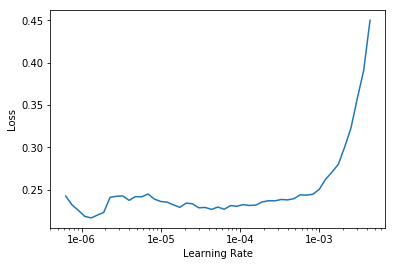how to unfreeze model and fit with a specific range of lr with epochs

#### how to unfreeze model and fit with a specific range of lr with epochs

``````learn.unfreeze()
learn.fit_one_cycle(2, max_lr=slice(1e-6,1e-4))
``````

Total time: 00:53

epoch train_loss valid_loss error_rate
1 0.242544 0.208489 0.067659
2 0.206940 0.204482 0.062246

That’s a pretty accurate model!

## Training: resnet50

what is the difference between resnet34 and resnet50

#### what is the difference between resnet34 and resnet50

Now we will train in the same way as before but with one caveat: instead of using resnet34 as our backbone we will use resnet50 (resnet34 is a 34 layer residual network while resnet50 has 50 layers. It will be explained later in the course and you can learn the details in the resnet paper).

why use larger model and image to train with smaller batch size?

#### why use larger model and image to train with smaller batch size?

Basically, resnet50 usually performs better because it is a deeper network with more parameters. Let’s see if we can achieve a higher performance here. To help it along, let’s us use larger images too, since that way the network can see more detail. We reduce the batch size a bit since otherwise this larger network will require more GPU memory.

how to create an ImageDatabunch with re and setting image size and batch size?

#### how to create an ImageDatabunch with re and setting image size and batch size?

``````data = ImageDataBunch.from_name_re(path_img,
fnames,
pat,
ds_tfms=get_transforms(),
size=299,
bs=bs//2).normalize(imagenet_stats)
``````
how to create an CNN model with this data?

#### how to create an CNN model with this data?

``````learn = create_cnn(data, models.resnet50, metrics=error_rate)
``````
find and plot the loss-lr relation

#### find and plot the loss-lr relation

``````learn.lr_find()
learn.recorder.plot()
``````
``````LR Finder complete, type {learner_name}.recorder.plot() to see the graph.
``````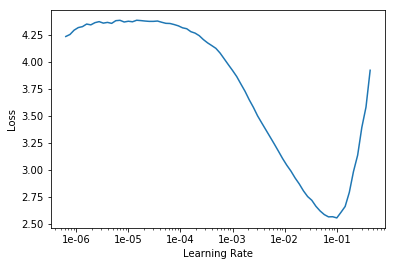how to fit the model 8 epochs

#### how to fit the model 8 epochs

``````learn.fit_one_cycle(8)
``````
``````Total time: 06:59
epoch  train_loss  valid_loss  error_rate
1      0.548006    0.268912    0.076455    (00:57)
2      0.365533    0.193667    0.064953    (00:51)
3      0.336032    0.211020    0.073072    (00:51)
4      0.263173    0.212025    0.060893    (00:51)
5      0.217016    0.183195    0.063599    (00:51)
6      0.161002    0.167274    0.048038    (00:51)
7      0.086668    0.143490    0.044655    (00:51)
8      0.082288    0.154927    0.046008    (00:51)
``````
how to save the model with a different name

#### how to save the model with a different name

``````learn.save('stage-1-50')
``````

It’s astonishing that it’s possible to recognize pet breeds so accurately! Let’s see if full fine-tuning helps:

how to unfreeze and fit with a specific range for 3 epochs

#### how to unfreeze and fit with a specific range for 3 epochs

``````learn.unfreeze()
learn.fit_one_cycle(3, max_lr=slice(1e-6,1e-4))
``````
``````Total time: 03:27
epoch  train_loss  valid_loss  error_rate
1      0.097319    0.155017    0.048038    (01:10)
2      0.074885    0.144853    0.044655    (01:08)
3      0.063509    0.144917    0.043978    (01:08)
``````
how to go back to previous model

#### how to go back to previous model

``````learn.load('stage-1-50');
``````
how to get classification model interpretor

#### how to get classification model interpretor

``````interp = ClassificationInterpretation.from_learner(learn)
``````
how to print out the most confused categories with minimum count

#### how to print out the most confused categories with minimum count

``````interp.most_confused(min_val=2)
``````
``````[('american_pit_bull_terrier', 'staffordshire_bull_terrier', 6),
('Bengal', 'Egyptian_Mau', 5),
('Bengal', 'Abyssinian', 4),
('boxer', 'american_bulldog', 4),
('Ragdoll', 'Birman', 4),
('Egyptian_Mau', 'Bengal', 3)]
``````

## Other data formats

how to get MNIST_SAMPLE dataset

#### how to get MNIST_SAMPLE dataset

``````path = untar_data(URLs.MNIST_SAMPLE); path
``````
``````PosixPath('/home/ubuntu/course-v3/nbs/dl1/data/mnist_sample')
``````
how to set flip false for transformation

#### how to set flip false for transformation

``````tfms = get_transforms(do_flip=False)
``````
how to create ImageDataBunch from folders

#### how to create ImageDataBunch from folders

``````data = ImageDataBunch.from_folder(path, ds_tfms=tfms, size=26)
``````
how to print out image examples from a batch

#### how to print out image examples from a batch

``````data.show_batch(rows=3, figsize=(5,5))
``````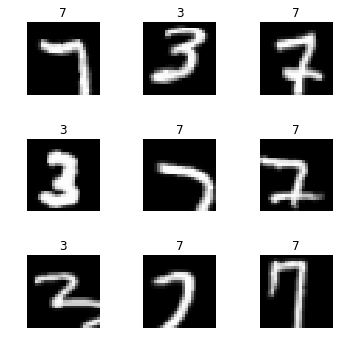how to create a cnn with resnet18 and accuracy as metrics

#### how to create a cnn with resnet18 and accuracy as metrics

``````learn = create_cnn(data, models.resnet18, metrics=accuracy)
``````
how to fit 2 epocs

#### how to fit 2 epocs

``````learn.fit(2)
``````
``````Total time: 00:23
epoch  train_loss  valid_loss  accuracy
1      0.116117    0.029745    0.991168  (00:12)
2      0.056860    0.015974    0.994603  (00:10)
``````
how to read a csv with pd

#### how to read a csv with pd

``````df = pd.read_csv(path/'labels.csv')
``````
how to read first 5 lines

#### how to read first 5 lines

``````df.head()
``````
.dataframe tbody tr th:only-of-type { vertical-align: middle; }
``````.dataframe tbody tr th {
vertical-align: top;
}

text-align: right;
}
``````
name label
0 train/3/7463.png 0
1 train/3/21102.png 0
2 train/3/31559.png 0
3 train/3/46882.png 0
4 train/3/26209.png 0
how to create ImageDataBunch with csv

#### how to create ImageDataBunch with csv

``````data = ImageDataBunch.from_csv(path, ds_tfms=tfms, size=28)
``````
how to print out images from a batch and classes

#### how to print out images from a batch and classes

``````data.show_batch(rows=3, figsize=(5,5))
data.classes
``````
``````[0, 1]
``````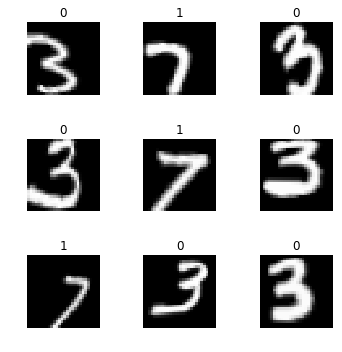how to create ImageDataBunch from df

#### how to create ImageDataBunch from df

``````data = ImageDataBunch.from_df(path, df, ds_tfms=tfms, size=24)
data.classes
``````
``````[0, 1]
``````
how to create file path object into a list from df

#### how to create file path object into a list from df

``````fn_paths = [path/name for name in df['name']]; fn_paths[:2]
``````
``````[PosixPath('/home/ubuntu/course-v3/nbs/dl1/data/mnist_sample/train/3/7463.png'),
PosixPath('/home/ubuntu/course-v3/nbs/dl1/data/mnist_sample/train/3/21102.png')]
``````
how to create ImageDataBunch from re

#### how to create ImageDataBunch from re

``````pat = r"/(\d)/\d+\.png\$"
data = ImageDataBunch.from_name_re(path, fn_paths, pat=pat, ds_tfms=tfms, size=24)
data.classes
``````
``````['3', '7']
``````
how to create ImageDataBunch from name function with file path list

#### how to create ImageDataBunch from name function with file path list

``````data = ImageDataBunch.from_name_func(path, fn_paths, ds_tfms=tfms, size=24,
label_func = lambda x: '3' if '/3/' in str(x) else '7')
data.classes
``````
``````['3', '7']
``````
how to create a list of labels from list of file path

#### how to create a list of labels from list of file path

``````labels = [('3' if '/3/' in str(x) else '7') for x in fn_paths]
labels[:5]
``````
``````['3', '3', '3', '3', '3']
``````
how to create an ImageDataBunch from list

#### how to create an ImageDataBunch from list

``````data = ImageDataBunch.from_lists(path, fn_paths, labels=labels, ds_tfms=tfms, size=24)
data.classes
``````
``````['3', '7']
``````
4 Likes